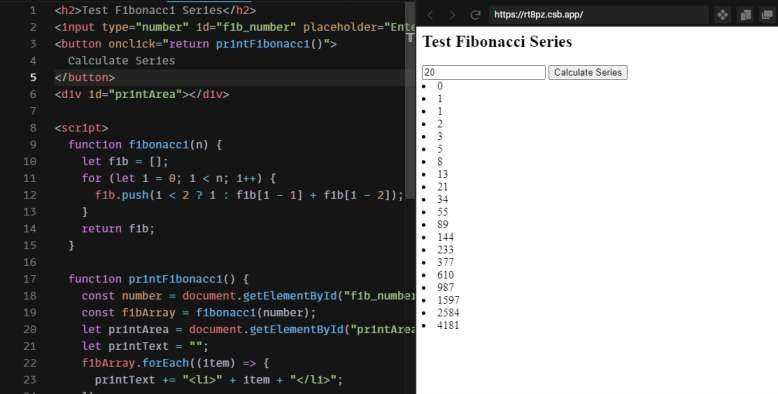Categories - JavaScript Tags - JavaScript   Maniruzzaman Akash   2 years ago   859   1 minute   0

# Fibonacci Series Algorithm with printing in JavaScript Line by Line Code Example

Hello Everyone, Today, we're going to print fibonacci series text's in browser.

Let's check first fibonacci series alrorithm -

It's very simple -

Work with Javascript for this Fibonacci Series:

``````function fibonacci(n) {
let fib = [];
for (let i = 0; i < n; i++) {
fib.push(i < 2 ? i : fib[i - 1] + fib[i - 2]);
}
return fib;
}

console.log("Fibonacci Series:", fibonacci(20));``````

Let's solve this fibonacci series problem line by line in javascript-

1. We created a `fibonacci`() function
2. Initialize the `fib[]` array as empty.
3. Loop throw this number. i-n
1. If i is less than 2, i = fibonacci number
2. If is is greater than or equal 2, `fib = fib[i-1] + fib[i-2]`

That's it. Basically algorithm is very simple.

fib = fib[i-1] + fib[i-2]

Now, let's make a demo to show this fibonacci series in browser -

So, check this fibonacci series problem in javascript and let me know if any more issues happen with Fibonacci series problem of javascript.